1. /
2. CBSE
3. /
4. Class 07
5. /
6. Mathematics
7. /
8. NCERT Solutions for Class...

# NCERT Solutions for Class 7 Maths Exercise 6.1### myCBSEguide App

Download the app to get CBSE Sample Papers 2023-24, NCERT Solutions (Revised), Most Important Questions, Previous Year Question Bank, Mock Tests, and Detailed Notes.

NCERT solutions for Maths Triangles and its Properties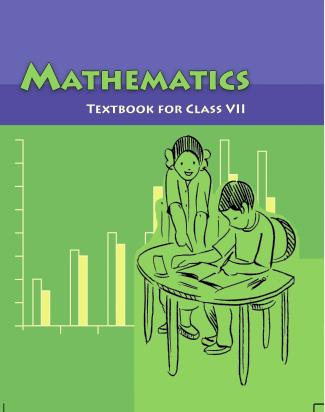## NCERT Solutions for Class 7 Maths Triangles and its Properties

Class –VII Mathematics (Ex. 6.1)

Question 1.In {tex}\Delta {/tex}PQR, D is the mid-point of {tex}\overline {{\text{QR}}} .{/tex}

{tex}\overline {{\text{PM}}} {/tex} is _______________

PD is ________________

Is QM = MR ?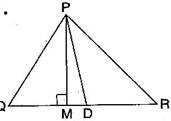Given: QD = DR

{tex}\therefore {/tex} {tex}\overline {{\text{PM}}} {/tex} is altitude.

PD is median.

No, QM {tex} \ne {/tex} MR as D is the mid-point of QR.

Question 2.Draw rough sketches for the following:

1. In {tex}\Delta {/tex}ABC, BE is a median.
2. In {tex}\Delta {/tex}PQR, PQ and PR are altitudes of the triangle.
3. In {tex}\Delta {/tex}XYZ, YL is an altitude in the exterior of the triangle.

(a) Here, BE is a median in {tex}\Delta {\text{ABC}}{/tex} and AE = EC.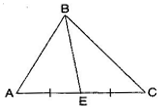(b) Here, PQ and PR are the altitudes of the {tex}\Delta {/tex}PQR and RP {tex} \bot {/tex} QP.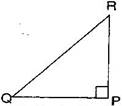(c) YL is an altitude in the exterior of {tex}\Delta {/tex}XYZ.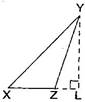Question 3.Verify by drawing a diagram if the median and altitude of a isosceles triangle can be same.

Isosceles triangle means any two sides are same.

Take {tex}\Delta {\text{ABC}}{/tex} and draw the median when AB = AC.

AL is the median and altitude of the given triangle.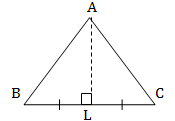## NCERT Solutions for Class 7 Maths Exercise 6.1

NCERT Solutions Class 7 Maths PDF (Download) Free from myCBSEguide app and myCBSEguide website. Ncert solution class 7 Maths includes text book solutions from Class 7 Maths Book . NCERT Solutions for CBSE Class 7 Maths have total 15 chapters. 7 Maths NCERT Solutions in PDF for free Download on our website. Ncert Maths class 7 solutions PDF and Maths ncert class 7 PDF solutions with latest modifications and as per the latest CBSE syllabus are only available in myCBSEguide.

## CBSE app for Students

To download NCERT Solutions for Class 7 Maths, Social Science Computer Science, Home Science, Hindi English, Maths Science do check myCBSEguide app or website. myCBSEguide provides sample papers with solution, test papers for chapter-wise practice, NCERT solutions, NCERT Exemplar solutions, quick revision notes for ready reference, CBSE guess papers and CBSE important question papers. Sample Paper all are made available through the best app for CBSE students and myCBSEguide website.Test Generator

Create question paper PDF and online tests with your own name & logo in minutes.myCBSEguide

Question Bank, Mock Tests, Exam Papers, NCERT Solutions, Sample Papers, Notes

### 3 thoughts on “NCERT Solutions for Class 7 Maths Exercise 6.1”

1. Very useful for children to study and understand

2. wow you are inteligent riya ok

3. It was very useful . thank you so much